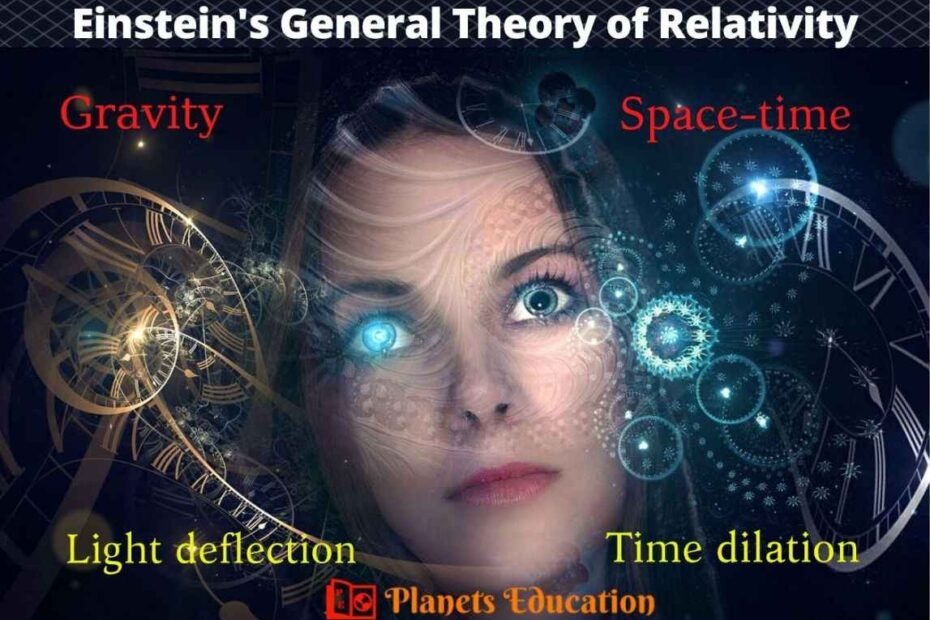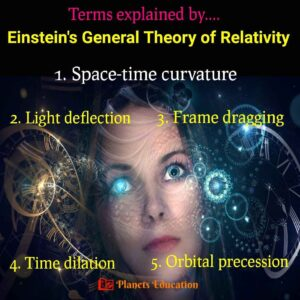# General theory of relativity by Einstein: ExplainedThe general theory of relativity by Einstein is also known as “general relativity”. Einstein’s “theory of relativity” is classified into two categories: 1. General theory of relativity, 2. Special theory of relativity.

The general relativity theory explains the geometrical concept of gravity in physics and was published by Albert Einstein in 1915. Whereas the special relativity theory explains the phenomenon of physics in the non-accelerating frame of reference and Albert Einstein published it in 1905.”

### Why did Einstein need to develop the general theory of relativity?

Sir Isaac Newton gave the law of gravity and stated that “every particle of the universe attracts every other particle with a force which is directly proportional to the product of their masses and inversely proportional to the square of their center to center distance”.

F=G×m1×m2/r2

Where F is the gravitational force between two objects and G is the gravitational constant

The gravitational law of Newton is applicable for objects moving slowly as compared to light. It violates the special theory of relativity. Special relativity theory is limited to the physics law for the non-accelerating frame (inertial frame of reference) that is the body is at rest or moving with a constant velocity.

Theory of special relativity neglect the effect of gravity on the physics laws, including the laws of motion. According to special relativity theory speed of light is constant in all frames of reference (neglecting the effect of gravity).

Einstein’s theory of relativity shows the meaning that everything is relative, that’s why it is called relativity theory. Special relativity only for special cases like a non-accelerating frame or frame of reference would be constant. So there is a need to publish a theory that can support an accelerating frame and with changing motion, and this is called Einstein’s general theory of relativity. In this theory frame of reference changes with respect to other frames while considering the effect of gravity.

## Principle of General Relativity

Einstein’s general relativity theory was superior to Newton’s law of gravity, but Newtonian gravity law is being continuously used in many applications for the approximation effect of gravity.

The basic principle of using the general theory of relativity is for finding a very accurate value of gravitational effect on any object in the universe. Newtonian gravity describes its effect on the force, whereas one of the main facts of general relativity theory is that it applies to the space-time curvature.Space-time curvature: Near to the Sun and Earth

This relativity theory is precisely applicable to finding a gravitational effect on an object with a strong gravitational field, or objects with extreme mass or density. The orbital effect of planet mercury around the sun is explained using this theory, as Sun is very near and dense compare to mercury.

## Experimental Facts about Einstein’s General Relativity

Special relativity is valid for the inertial frame of reference or constant velocity or non-accelerating. But in our universe, everything is accelerating, and considering acceleration as a reference Einstein published the general theory of relativity after 10 years.

#### A thought experiment on free fall or acceleration

Before publishing the general relativity theory Albert Einstein did some research on gravity and acceleration. He did some thought experiments on the term “free falling”. Let’s see the thought experiments done by Einstein with an example: Suppose a person is floating into a lift that is also floating in space. If we draw the lift upside with an acceleration of 9.8 m/sec2. The person will collide with the base of the lift like it is happening on the earth. The person can not describe that he is on earth or in space. According to this experiment, Einstein concluded that acceleration is gravity.

#### A thought experiment on gravity

According to Newton, due to gravity objects are attached to each other and it also applies in the whole universe including our solar system. Newton also told that action of the gravity is instant. But Albert Einstein told that nothing happens in an instant and he brought up the term speed of light.

Here Einstein did another thought experiment, let’s see this with an example. What if our Sun disappears instantly from the solar system? According to Newton’s law, the earth would start moving in a straight line as there would not be any gravity of the sun to give it an orbital motion. But Einstein told that Earth must orbit for at least 8 minutes even after disappearing from the sun.

#### A thought experiment on space-time curvature

For understanding gravity, Einstein researched gravity with the term space-time. According to him, space-time is like a fabric sheet. He described that if we put mass on the fabric sheet then it will form a curve shape. Similarly, objects with mass give shape to space-time and the gravitational effect applies to space-time curvature. The objects with more mass will curve the fabric sheet more. Similarly, the space-time curvature or gravity will be more if the object has more mass.A fabric sheet representing space-time curvature

## Consequences of the general theory of relativity

Einstein’s general theory of relativity gave many consequences in modern physics and still, scientists are researching it to conclude more. The following are the physical consequences of general relativity theory:Consequences of the general theory of relativity

### 1. Gravitational time dilation

Einstein’s theory showed that gravity also affects time and this phenomenon is called gravitational time dilation. At the place gravity is low, time will be faster. Whereas if the gravity is more time will run slow. This effect is tested by Einstein’s general relativity.

According to this effect, clocks with different gravitational potentials or different altitudes will show different times. If the gravitational potential difference is too high between two places, this effect would be a considerable point. Whereas the time dilation with the gravitational potential difference on earth can be negligible.

The earth’s core is 2.5 years younger than its surface the relative to earth’s age in this all billion of years. This is because the core of the earth has more gravity and time run slowly there. We can see a considerable gravitational time dilation in the black hole where gravity is enormous.

• Must Check:-

### 2. Light bending or deflection

Light bends due to space-time curvature. For example: in our solar system sun is the most massive and it creates more space-time curvature and that’s how it bends the light of stars that are the backside of the sun. For proving it Einstein did an experiment on the day of the solar eclipse. According to the general theory of relativity, the path of light will follow the space-time curvature and will deflect the light path at the curved place.

### 3. Orbital effects or precession

The precession of the interstellar rotating body is a continuous change in its orbital axis. The orbital path of our planets around the sun does not follow the exact at each time. The planet Mercury has the most elliptical orbit and it was not following Newton’s theory of gravity. In the change of the precession rate of Mercury, the orbit is predicted accurately with the help of the theory of general relativity.

### 4. Frame dragging

According to Newton’s law of motion, the gravitational field of a body depends on its mass, not on its rotation. Whereas Einstein’s relativity theory predicts that the rotation of a massive body will distort the space-time metrics. This frame-dragging effect is also known as gravitomagnetism and can drag around the neighborhood of space-time vicinity.  The frame-dragging phenomenon was derived from general relativity and happens in the rotational black hole.

So, these were some explanations about the general theory of relativity and some relevant terms. Hope you have liked reading it. Check the below-related posts for more interesting planets and space information.

Related articles:-

## 3 thoughts on “General theory of relativity by Einstein: Explained”

1.If the speed of light is constant (speed being defined by distance traveled over time) but time itself passes at a different rate according to gravity, how can the interval for light to reach us from a distant star accurately measure distance? As the light travels through space-time it is subject to an ever changing gravity.

1.We can not measure the accurate distance of the distant objects. The speed of light is constant according to General relativity theory but the universe is accelerating. We have some methods to measure the distance of the astronomical object. If it is too far we add the accelerated term and “ESTIMATE” the distance. I hope you can get it.

2.We can not measure the accurate distance of the distant objects. The speed of light is constant according to General relativity theory but the universe is accelerating. We have some methods (one is parallax) to measure the distance of the astronomical object. If it is too far we add the accelerated term and “ESTIMATE” the distance. I hope you can get it.